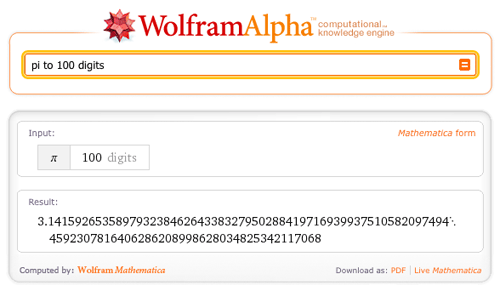AUTHOR PAGEEric Weisstein
Blog Posts from this author:
October 3, 2011–

As most Wolfram|Alpha blog readers know, the engine behind the Wolfram|Alpha computational knowledge engine is Wolfram Research’s powerful mathematics and computation software, Mathematica. Ironically, while Wolfram|Alpha contains thousands of datasets on diverse and sundry subject areas, until very recently, its computable knowledge of the Mathematica language itself has been somewhat limited. More »

August 5, 2011–

Game theory is a rich branch of mathematics that deals with the analysis of games, where, mathematically speaking, a “game” can be defined as a conflict involving gains and losses between two or more opponents who follow formal rules.

Mathematical games can be very simple, such as the game of chicken (which is not recommended in practice):

March 14, 2011–

Yes, it is once again the time of the year when the mathematically inclined gather together to celebrate Pi Day…and, in the process, swap trivia of note on everyone’s (including Wolfram|Alpha’s) favorite number.

There have been no shortage of blog posts already written on the subject; see, for example, last year’s “Pi Day in Wolfram|Alpha” (or the Wolfram Blog Pi Day posts from 2008 or 2010). As already hinted at in last year’s blog, one would expect the pi to be ubiquitous in a computational knowledge engine—and so it is. Therefore, at the risk of beating a proven transcendental constant to death, this year we offer a few (well, OK: more than a few) additional pi-related esoterica courtesy of Wolfram|Alpha.
More »

January 28, 2011–

It is immediately clear to anyone who has used the site that Wolfram|Alpha knows a lot about mathematics. When computing integrals, sums, statistics, properties of mathematical objects, or a myriad of other mathematical and mathematics-related problems, it typically returns an extensive and exhaustively complete result. Which is of course not surprising, given that Wolfram|Alpha has the entire power and knowledge of Mathematica behind it, especially when combined with the fact that this native “smarts” is further augmented with large amounts of curated data and customized processing.

However, many visitors to the site have noted in the past that Wolfram|Alpha had relatively little computable knowledge about mathematical terms themselves, a state of affairs in contrast to its knowledge of words in the English language, and perhaps surprising in light of the existence of another Wolfram site devoted to the definition and description of terms in mathematics, namely MathWorld.

As readers of MathWorld likely already know, the entire MathWorld website is written and built using Mathematica. It has therefore been possible to programmatically process the entire 13,000+ entries comprising MathWorld into the native data format of Wolfram|Alpha, thus exposing its content in more computable form.

As an example of the sort of new knowledge this confluence brings to Wolfram|Alpha, consider the input “Lorenz attractor”. In the past, this would simply bring up a Wolfram|Alpha future topic page.

With the incorporation of MathWorld content, the default parse now goes to a description of the attractor, complete with an illustrative figure and some helpful typeset equations: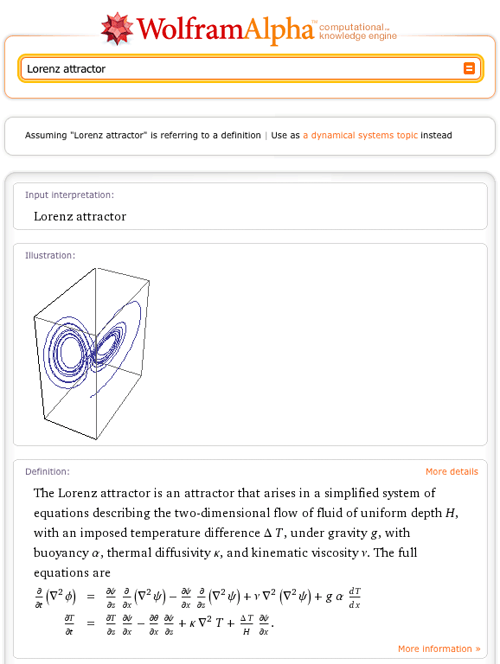December 17, 2010–

Wolfram|Alpha already contains many extensive collections of mathematical data, including curves, surfaces, graphs, knots, and polyhedra. However, one type of object we had not systematically incorporated until recently was the class of plane geometric figures technically known as laminae: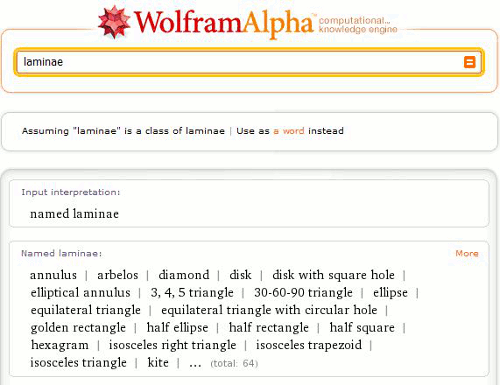Most people (including the subset of small people who play with sorting toys such as the one illustrated below) are familiar with a number of laminae. A lamina is simply a bounded (and usually connected) region of the Euclidean plane. In the most general case, it has a surface density function ?(x, y) as a function of x– and y-coordinates, but with ?(x, y) = 1 in the simplest case.Examples of laminae, some of which are illustrated above, therefore include the disk (i.e., filled circle), equilateral triangle, square, trapezoid, and 5-point star. In the interest of completeness, it might be worth mentioning that laminae are always “filled” objects, so the ambiguity about whether the terms “polygon”, “square”, etc. refer to closed sets of line segments or those segments plus their interiors does not arise for laminae.
More »

May 4, 2010–

As we all know by now, Wolfram|Alpha is a computational knowledge engine. That means not only should it be able to do computations on a wide variety of topics, but also that it needs detailed knowledge of the names and salient properties of a wide variety of entities that are commonly encountered in human inquiry and discourse.

This is obvious in the case of classes of objects that fit neatly into curated data collections, such as mathematical surfaces (e.g., Möbius strip), countries of the world (e.g., New Zealand), chemicals (e.g., caffeine), and so forth.

What is perhaps slightly less obvious is just how much knowledge needs to be encoded to have a reasonable “understanding” of almost any named result in math and the sciences. For example, most people (including non-mathematicians) have heard of Fermat’s last theorem and therefore would rightly expect Wolfram|Alpha to be able to say something sensible about it.  And as one of my other hats involves writing the online encyclopedia of math known as MathWorld, which is hosted and sponsored by Wolfram Research, putting this information into Wolfram|Alpha naturally fell to me. So, for the past several months, I have been attempting to gradually build up Wolfram|Alpha’s knowledge base on named results in math and physics.

The screenshot below shows what Wolfram|Alpha now returns for Fermat’s last theorem: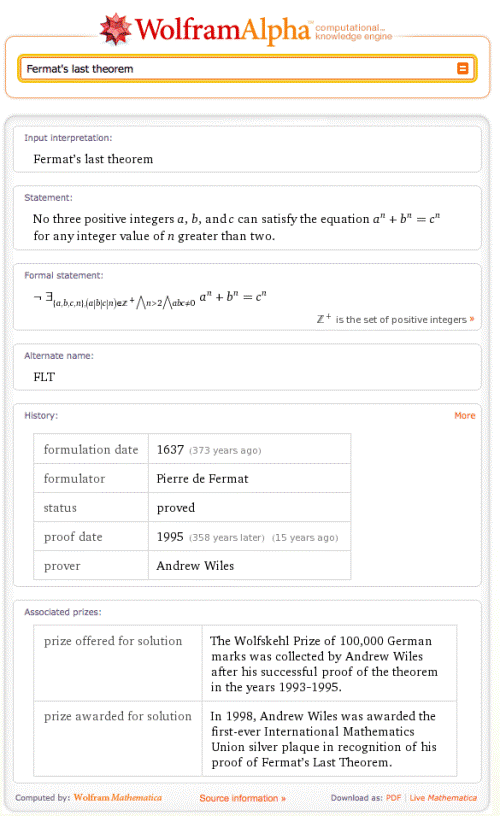As you can see, Wolfram|Alpha begins by giving you the standard name for the result in question, followed by a clearly worded (or at least as clearly worded as could be managed in the marginal space available 😉 ) plain English statement of the result. Next, at least in cases where it is possible to do so, a mathematically precise “formal statement” of the result is given. This is followed by any common alternate names the result might have, a listing of historical information, and finally an enumeration of prizes associated with it (where relevant). More »

March 12, 2010–

In my blog post last month, I wrote about Valentine’s Day in Wolfram|Alpha. Strangely, we received a number of comments indicating that the computational power of Wolfram|Alpha was not always sufficient to melt the hearts of some non-mathematically inclined sweethearts of the world. But not to fear; I have decided to persist undeterred in spite of that disappointing and surprising news, now that we’re on the verge of another holiday (and a more inherently mathematical one).

The holiday in question is Pi Day. As with a large number of other holidays, simply typing its name (in this case, “pi day”) into Wolfram|Alpha gives you basic calendrical information about it:Now, because Wolfram|Alpha users are both intelligent and discriminating, all of you have I’m sure already noticed that when the digits in the date 3/14 (March 14 in the United States style for dates—a bit more about this later) are run together with a decimal place between, the result is 3.14. And that that decimal expansion is connected with a certain famous mathematical constant given by the ratio of the circumference of a circle to its diameter. And that little fact explains why Pi Day is celebrated on the 14th of each March. More »

February 12, 2010–

Valentine’s Day is special to sweethearts around the world. While Wolfram|Alpha can’t come close to replacing a thoughtful card or gourmet box of chocolates, there are a surprisingly large number of things related to Valentine’s Day (and in particular, to its central icon) that Wolfram|Alpha can compute.

Let’s start with the holiday itself. Just typing in “valentine’s day” gives the expected calendrical information, from which we learn that Valentine’s Day falls on a Sunday this year. For the procrastinators among us, we can also find out how many days we have remaining to acquire an appropriate token of affection for our loved one (or by how many days we’ve already blown our chance). Wolfram|Alpha also shows various other useful data, including the interesting fact that Valentine’s Day coincides with Chinese New Year this year.

While Wolfram|Alpha can’t (yet) tell you how many calories are in your box of holiday chocolates or package of Valentine’s Day Sweethearts candy, there are plenty of computational objects related to that most-famous Valentine’s Day icon—the heart—that it can tell you something interesting and/or useful about. For instance, do you know the average weight of a human heart? The typical resting heart rate? The Unicode point for the heart symbol character? Or perhaps you’ve forgotten the ASCII keystrokes needed to insert a love emoticon at the end of an email to your Sweet Baboo?

On the mathematical side, typing in “heart curve” gives you a number of mathematical curves resembling the heart shape. The default (and probably most famous) of these is the cardioid, whose name after all means “heart-shaped” in Latin (and about which we all have fond memories dating back to our introductory calculus courses):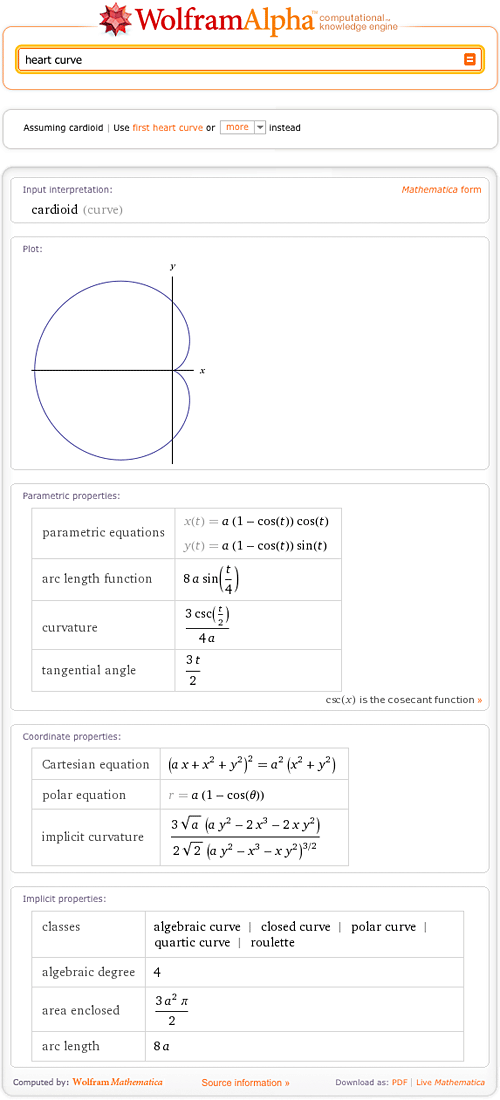A curve more closely resembling the conventional schematic (if not physiological) heart shape is the so-called “first heart curve“, which is an algebraic curve described by a beautifully simple sextic Cartesian equation:If you don’t care for any of the heart curves Wolfram|Alpha knows about (or even if you do), you’re also of course also free to experiment with your own. For example, a particularly attractive curve can be obtained using the relatively simple input “polar plot 2 – 2 sin t + sin t sqrt (abs(cos t))/(sin t + 1.4)“. More »

May 19, 2009–

Every aspect of Wolfram|Alpha has been thought through in great detail. Its logo is no exception.

As a tip of the hat to the vast and powerful computational engine that powers Wolfram|Alpha, a natural place to start brainstorming for an appropriate logo was in Mathematica itself. And this is where I, geometry enthusiast and the developer of the PolyhedronData computational data collection, came into the picture.

As many of you may know, Mathematica‘s logo is a three-dimensional polyhedron affectionately called “Spikey.” In its original (Version 1) form, Spikey consisted of the spiked solid obtained from an icosahedron (the regular 20-faced solid that is one of the five Platonic solids) with regular tetrahedra (triangular pyramids) affixed to its faces.More »

Tags:
May 13, 2009–

Some of you have asked whether you’ll be able to use Wolfram|Alpha for challenging math. Of course!

Remember your old friend pi?